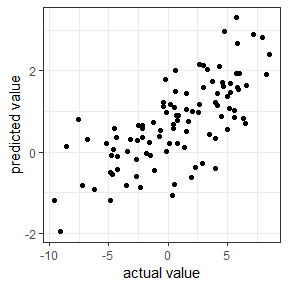The glmaag package: adaptive mixture network regularized generalized linear models

2019-05-09

This package allows users to run adaptive mixture network regularized generalized linear models (Gaussian, logistic, and Cox regression). The model looks like $argmax_{\beta}\left\{ \frac{1}{2n}l\left(\beta\right)-\lambda_{1}\sum_{i=1}^{p}w_{i}\left|\beta_{i}\right|-\frac{\lambda_{2}}{2}\left|\beta\right|^{T}L\left|\beta \right|\right\}.$ Usually we don’t know the proper values for $$\lambda_1$$ and $$\lambda_2$$. Here we provided two ways to tune the parameters: cross validation and stability selection. To solve this problem we need a structure of the network. For example

library(glmaag)
## Registered S3 methods overwritten by 'ggplot2':
##   method         from
##   [.quosures     rlang
##   c.quosures     rlang
##   print.quosures rlang
## Registered S3 methods overwritten by 'pROC':
##   method    from
##   print.roc huge
##   plot.roc  huge
data(sampledata)
data(L0)
y <- sampledata\$Y_Gau
x <- as.matrix(sampledata[, -(1:3)])
mod <- cv_glmaag(y, x, L0)

However, in practice it is very common that we don’t know the network structure in advance. To address this issue, we can preestimate the network structure

L1 <- getS(x)

Or simply we ran the following code

mod <- cv_glmaag(y, x)
## Use estimated network

If we don’t specify any network structure, function cv_glmaag can estimate the network automaticaaly.If the user wants to use adaptive elastic net penalty rather than adaptive network penalty, he or she can still use our package with the following statement

mod <- cv_glmaag(y, x, est = F)
## Use elastic net

If we have two network structures but not know which one to use, we can tune the two network by cross validation prdiction

L <- tune_network(y, x, L0, L1)@est

If we have a known network and want to mix the known network with a network estimated from the data, we can run

mod <- cv_glmaag(y, x, L0, tune = T)

If the user has enough time and want to have a better feature selection accuracy, he or she may perform stablity selection rather than cross validation. Usually we need 100 subsets, but here we do 5 subsets as an example.

mod <- ss_glmaag(y, x, L0, nsam = 5)

If we want to get the coeffcients of the model, predict data and evaluate the model we can run the following codes

coeffi <- coef(mod)
ypre <- predict(mod)
evaluate(ypre, y)
##        MAE        MSE    Pearson   Spearman
##  2.9466298 12.7073765  0.7101784  0.7197480
evaluate_plot(ypre, y)Now the users learn the basic operations of this package. If the user wants a visualized version, one can run the shiny app

runtheExample()

Enjoy the package!